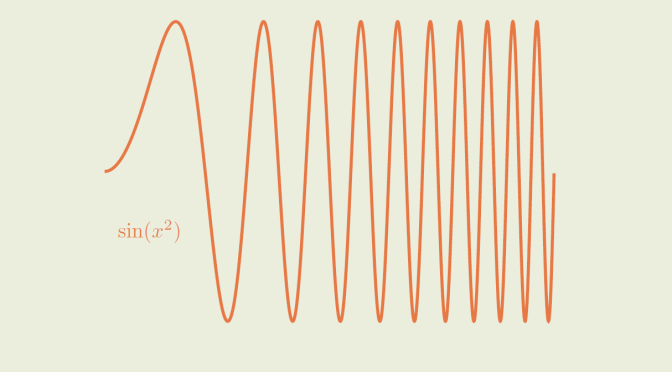# Continuity versus uniform continuity

We consider real-valued functions.

A real-valued function $$f : I \to \mathbb R$$ (where $$I \subseteq$$ is an interval) is continuous at $$x_0 \in I$$ when: $(\forall \epsilon > 0) (\exists \delta > 0)(\forall x \in I)(\vert x- x_0 \vert \le \delta \Rightarrow \vert f(x)- f(x_0) \vert \le \epsilon).$ When $$f$$ is continuous at all $$x \in I$$, we say that $$f$$ is continuous on $$I$$.

$$f : I \to \mathbb R$$ is said to be uniform continuity on $$I$$ if $(\forall \epsilon > 0) (\exists \delta > 0)(\forall x,y \in I)(\vert x- y \vert \le \delta \Rightarrow \vert f(x)- f(y) \vert \le \epsilon).$

Obviously, a function which is uniform continuous on $$I$$ is continuous on $$I$$. Is the converse true? The answer is negative.

### An (unbounded) continuous function which is not uniform continuous

The map $\begin{array}{l|rcl} f : & \mathbb R & \longrightarrow & \mathbb R \\ & x & \longmapsto & x^2 \end{array}$ is continuous. Let’s prove that it is not uniform continuous. For $$0 < x < y$$ we have $\vert f(x)-f(y) \vert = y^2-x^2 = (y-x)(y+x) \ge 2x (y-x)$ Hence for $$y-x= \delta >0$$ and $$x = \frac{1}{\delta}$$ we get
$\vert f(x) -f(y) \vert \ge 2x (y-x) =2 > 1$ which means that the definition of uniform continuity is not fulfilled for $$\epsilon = 1$$.

For this example, the function is unbounded as $$\lim\limits_{x \to \infty} x^2 = \infty$$.

### A bounded continuous function which is not uniform continuous

Let’s consider $\begin{array}{l|rcl} g : & \mathbb R & \longrightarrow & \mathbb R \\ & x & \longmapsto & \sin(x^2) \end{array}$ $$g$$ is continuous on $$\mathbb R$$ as it is the function composition of two continuous functions. For $$k \in \mathbb N$$, we denote $$x_k=\sqrt{2 k \pi}$$ and $$y_k=\sqrt{2 k \pi + \frac{\pi}{2}}$$. We have
$g(y_k)-g(x_k)= \sin(2 k \pi+\frac{\pi}{2}) – \sin(2 k \pi)=1$ and
\begin{aligned} 0 \le y_k-x_k &= \sqrt{2 k \pi + \frac{\pi}{2}}-\sqrt{2 k \pi}\\ &= \frac{\pi}{2} \frac{1}{\sqrt{2 k \pi + \frac{\pi}{2}}+\sqrt{2 k \pi}}\\ &\le \frac{\pi}{4}\frac{1}{\sqrt{2 k \pi}} \end{aligned} The uniform continuity definition is not fulfilled for $$\epsilon=1$$ because $$\lim\limits_{k \to \infty} \frac{\pi}{4}\frac{1}{\sqrt{2 k \pi}}=0$$. Hence $$\lim\limits_{k \to \infty} y_k-x_k=0$$ while $$g(y_k)-g(x_k)=1$$ for all $$k \in \mathbb N$$.

To conclude, let’s recall that according to Heine-Cantor theorem, a function that is continuous on a closed interval $$I$$ is also uniform continuous. So, it is not possible to find an example as in previous paragraph for a function defined on a closed interval $$I$$.

The following exercise is left to the reader interested in uniform continuity.

Exercise: A real-valued function defined and uniform continuous on $$[0,1)$$ is bounded and has a limit at $$1$$. Find counterexamples proving that both conclusions might be wrong for a continuous function.